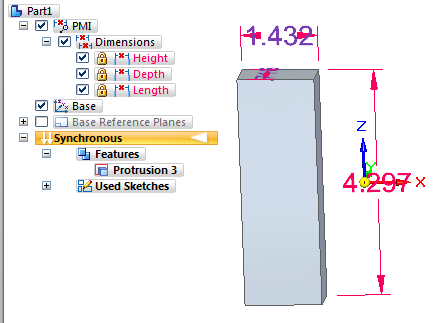VariablesVariables in Solid Edge are intuitively easy to use. As a former SolidWorks guy, Solid Edge Variables simplify things immensely (link values, equations, global variables, variables, etc in SW) and at the same time give you more power for controlling dimensions. Even in a synchronous part. Let’s have a look at the Variable Table. You can find the Variable Table on the Tools tab, in the Variables group.The first thing I notice about the table is that it allows you to control the Lock/Unlock condition of the dimensions right off the bat. It lists all the dimensions in the part, and their Lock/Unlock state, and lets you change the names, state and even the dimension value right from the Variable table.

The Rule column is read only, and tells you what is driving the dimension. It could be a Formula such as = Length + 4 or a Limit such as (1;8), which would limit the value to between 1 and 8. It’s very straight forward and makes named dimensions very easy to work with.Beyond the Variables Table, the Pathfinder also gets involved as well as the PMI display on the parts. First, notice that this part is Synchronous, and it is using variables. That means that Synchronous parts are truly parametric for those of you who had any remaining doubt.

Notice that the Dimension area of the Pathfinder gives names to the dimensions. These are the variable names. You can toggle the display of dimension values or names by right clicking on a dimension in the Pathfinder, and making the selection from the list.

Also notice that the colors of the dimensions tell you the function of the dimension.

• Red = locked (can only be changed directly by the user)

• Blue = unlocked (can be changed by the system or by the user)

• Purple = driven by a Formula

Solid Edge will even tell you if you try to set up conflicting equations. Lets say for example that you have two dimensions on a part, Height and Diameter. You write two formulas as Height = Diameter * 2 and Diameter = Height / 2. While the formulas can be satisfied simultaneously, they are overconstrained and circular (A drives B and B drives A) because there is not a clear driving term. Solid Edge identifies this situation and will not allow you to actually create the second equation. SE gives you the error message “Variable Depth is driven and connot be used within related geometry to evaluate driving variable Diameter.” That’s reasonably easy to understand, I think.

Solid Edge also will warn you if you try to use an unlocked dimension to drive a formula. This prevents you from having too many sources driving the dimensions of your parts.

Overall, the Variable Table and the Pathfinder display are areas that I tink are very nicely developed. The functionality is practical and easy to figure out. One thing that might be added would be if you could click on dimensions to add them to the Forumula box, or maybe there could be a calculator keypad.

Topics